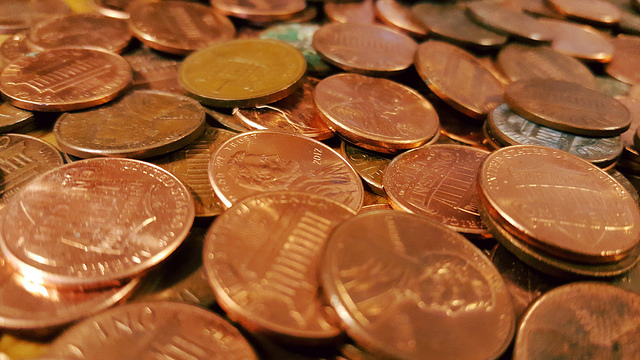# Balance Scale As A Mathematical Model

Student View (Opens in new window)# Lesson Overview

Students solve a classic puzzle about finding a counterfeit coin. The puzzle introduces students to the idea of a scale being balanced when the weight of the objects on both sides is the same and the scale being unbalanced when the objects on one side do not weigh the same as the objects on the other side.

# Key Concepts

The concept of an inequality statement can be modeled using an unbalanced scale. The context—weighing a set of coins in order to identify the one coin that weighs less than the others—allows students to manipulate the weight on either side of the scale. In doing so, they are focused on the relationship between two weights—two quantities—and whether or not they are equal.

# Goals and Learning Objectives

• Explore a balance scale as a model for an equation or an inequality.
• Introduce formal meanings of equality and inequality.